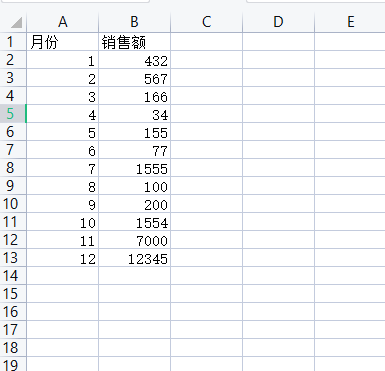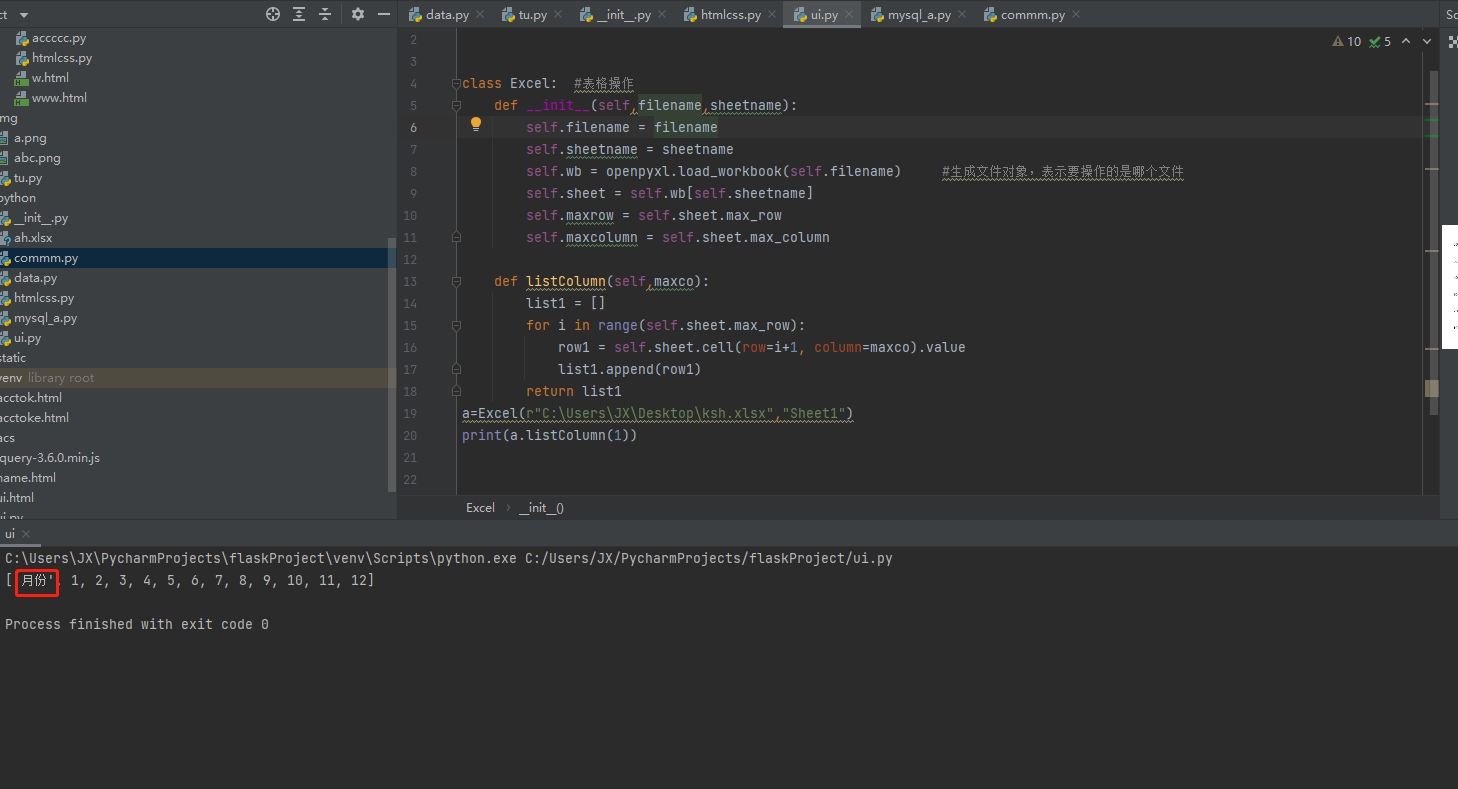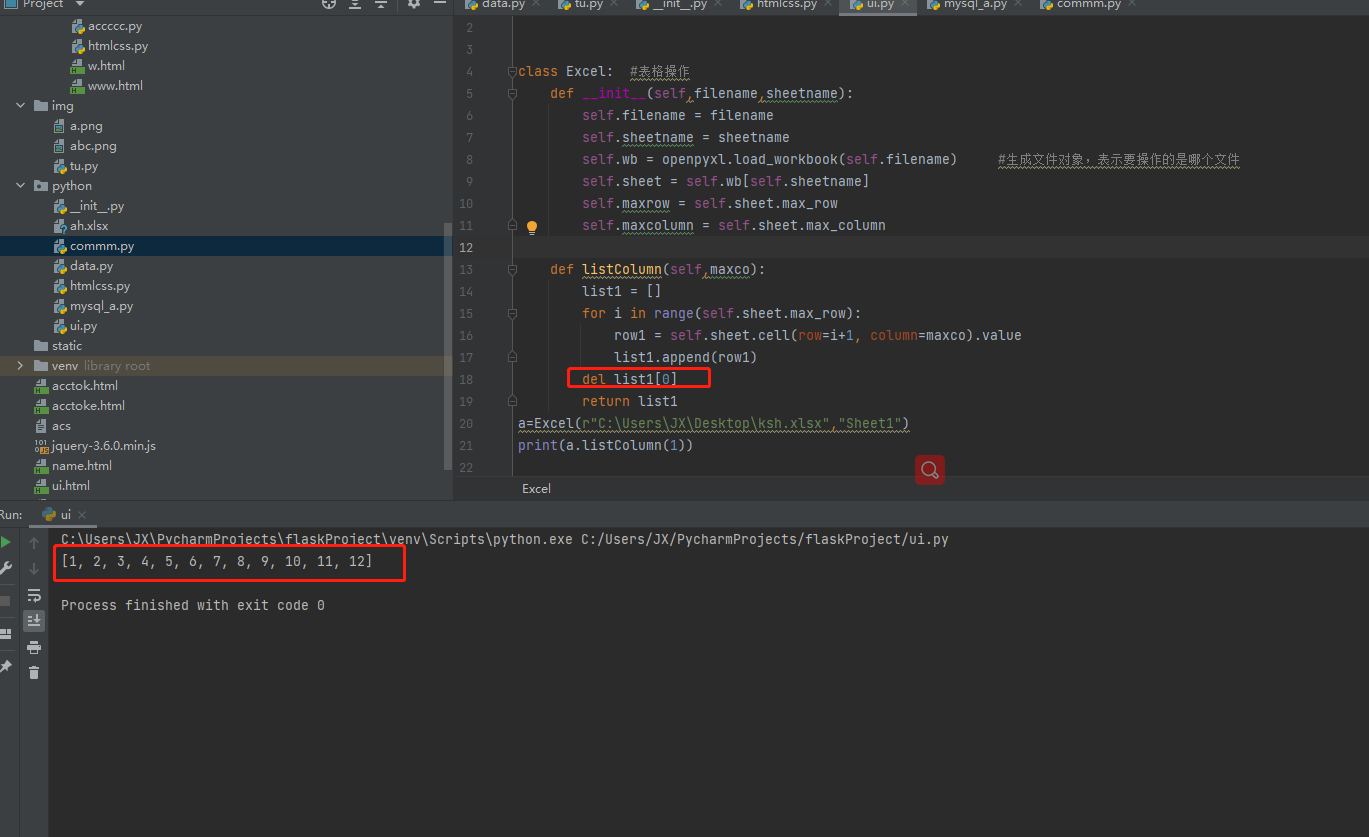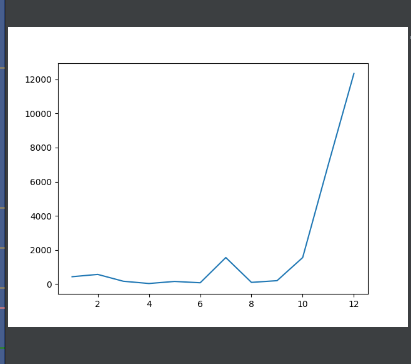## python生成可视化数据（matplotlib）# 一，python生成可视化数据

``````import matplotlib.pyplot as plt

x_data_csdn = ['2011', '2012', '2013', '2014', '2015', '2016', '2017']
y_data_csdn= [80,90,112,400,600,100,500]

plt.plot(x_data_csdn, y_data_csdn)
plt.show()``````

# 二，将表格的数据转换为x轴和y轴所需要的数据既然方法意见写好了 那我们就把数据导入到x轴和y轴里面吧

# 三，导入数据

``````import openpyxl
import matplotlib.pyplot as plt

class Excel:  #表格操作
def __init__(self,filename,sheetname):
self.filename = filename
self.sheetname = sheetname
self.sheet = self.wb[self.sheetname]
self.maxrow = self.sheet.max_row
self.maxcolumn = self.sheet.max_column

def listColumn(self,maxco):
list1 = []
for i in range(self.sheet.max_row):
row1 = self.sheet.cell(row=i+1, column=maxco).value
list1.append(row1)
del list1
return list1
x=Excel(r"C:\Users\JX\Desktop\ksh.xlsx","Sheet1").listColumn(1)  #定义x轴内容

y=Excel(r"C:\Users\JX\Desktop\ksh.xlsx","Sheet1").listColumn(2)  #定义y轴内容

x_data_csdn = x   #将x轴输入导入
y_data_csdn= y   #将y轴数据导入

plt.plot(x_data_csdn, y_data_csdn)
plt.show()``````